Search by Topic

Resources tagged with Interactivities similar to Substitution Cipher:

Filter by: Content type:
Age range:
Challenge level:

There are 106 results

Broad Topics > Information and Communications Technology > InteractivitiesSubstitution Cipher

Age 11 to 14 Challenge Level:

Find the frequency distribution for ordinary English, and use it to help you crack the code.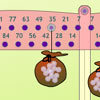Balancing 2

Age 11 to 14 Challenge Level:

Meg and Mo still need to hang their marbles so that they balance, but this time the constraints are different. Use the interactivity to experiment and find out what they need to do.Drips

Age 7 to 14 Challenge Level:

An animation that helps you understand the game of Nim.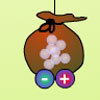Balancing 3

Age 11 to 14 Challenge Level:

Mo has left, but Meg is still experimenting. Use the interactivity to help you find out how she can alter her pouch of marbles and still keep the two pouches balanced.Cosy Corner

Age 11 to 14 Challenge Level:

Six balls are shaken. You win if at least one red ball ends in a corner. What is the probability of winning?Treasure Hunt

Age 7 to 14 Challenge Level:

Can you find a reliable strategy for choosing coordinates that will locate the treasure in the minimum number of guesses?Two's Company

Age 11 to 14 Challenge Level:

Seven balls are shaken. You win if the two blue balls end up touching. What is the probability of winning?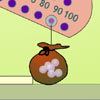Balancing 1

Age 11 to 14 Challenge Level:

Meg and Mo need to hang their marbles so that they balance. Use the interactivity to experiment and find out what they need to do.Right Angles

Age 11 to 14 Challenge Level:

Can you make a right-angled triangle on this peg-board by joining up three points round the edge?Isosceles Triangles

Age 11 to 14 Challenge Level:

Draw some isosceles triangles with an area of $9$cm$^2$ and a vertex at (20,20). If all the vertices must have whole number coordinates, how many is it possible to draw?Square Coordinates

Age 11 to 14 Challenge Level:

A tilted square is a square with no horizontal sides. Can you devise a general instruction for the construction of a square when you are given just one of its sides?Number Pyramids

Age 11 to 14 Challenge Level:

Try entering different sets of numbers in the number pyramids. How does the total at the top change?More Number Pyramids

Age 11 to 14 Challenge Level:

When number pyramids have a sequence on the bottom layer, some interesting patterns emerge...Stars

Age 11 to 14 Challenge Level:

Can you find a relationship between the number of dots on the circle and the number of steps that will ensure that all points are hit?Semi-regular Tessellations

Age 11 to 16 Challenge Level:

Semi-regular tessellations combine two or more different regular polygons to fill the plane. Can you find all the semi-regular tessellations?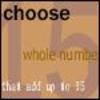Fifteen

Age 7 to 14 Challenge Level:

Can you spot the similarities between this game and other games you know? The aim is to choose 3 numbers that total 15.Estimating Angles

Age 7 to 14 Challenge Level:

How good are you at estimating angles?Excel Interactive Resource: Multiples Chain

Age 11 to 16 Challenge Level:

Use an interactive Excel spreadsheet to investigate factors and multiples.Got it Article

Age 7 to 14

This article gives you a few ideas for understanding the Got It! game and how you might find a winning strategy.Overlap

Age 11 to 14 Challenge Level:

A red square and a blue square overlap so that the corner of the red square rests on the centre of the blue square. Show that, whatever the orientation of the red square, it covers a quarter of the. . . .Excel Interactive Resource: Interactive Division

Age 11 to 16 Challenge Level:

Use an Excel to investigate division. Explore the relationships between the process elements using an interactive spreadsheet.Nine Colours

Age 11 to 16 Challenge Level:

Can you use small coloured cubes to make a 3 by 3 by 3 cube so that each face of the bigger cube contains one of each colour?Konigsberg Plus

Age 11 to 14 Challenge Level:

Euler discussed whether or not it was possible to stroll around Koenigsberg crossing each of its seven bridges exactly once. Experiment with different numbers of islands and bridges.Partitioning Revisited

Age 11 to 14 Challenge Level:

We can show that (x + 1)² = x² + 2x + 1 by considering the area of an (x + 1) by (x + 1) square. Show in a similar way that (x + 2)² = x² + 4x + 4Olympic Magic

Age 14 to 16 Challenge Level:

in how many ways can you place the numbers 1, 2, 3 … 9 in the nine regions of the Olympic Emblem (5 overlapping circles) so that the amount in each ring is the same?Tilted Squares

Age 11 to 14 Challenge Level:

It's easy to work out the areas of most squares that we meet, but what if they were tilted?Picturing Triangular Numbers

Age 11 to 14 Challenge Level:

Triangular numbers can be represented by a triangular array of squares. What do you notice about the sum of identical triangle numbers?Subtended Angles

Age 11 to 14 Challenge Level:

What is the relationship between the angle at the centre and the angles at the circumference, for angles which stand on the same arc? Can you prove it?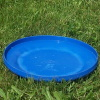Flippin' Discs

Age 11 to 14 Challenge Level:

Discs are flipped in the air. You win if all the faces show the same colour. What is the probability of winning?An Unhappy End

Age 11 to 14 Challenge Level:

Two engines, at opposite ends of a single track railway line, set off towards one another just as a fly, sitting on the front of one of the engines, sets off flying along the railway line...Square It

Age 11 to 16 Challenge Level:

Players take it in turns to choose a dot on the grid. The winner is the first to have four dots that can be joined to form a square.Excel Interactive Resource: Fraction Multiplication

Age 11 to 16 Challenge Level:

Use Excel to explore multiplication of fractions.Percentages for Key Stage 4

Age 14 to 16 Challenge Level:

A group of interactive resources to support work on percentages Key Stage 4.Excel Interactive Resource: Long Multiplication

Age 11 to 16 Challenge Level:

Use an Excel spreadsheet to explore long multiplication.Excel Interactive Resource: Equivalent Fraction Bars

Age 11 to 16 Challenge Level:

A simple file for the Interactive whiteboard or PC screen, demonstrating equivalent fractions.Excel Interactive Resource: Haitch

Age 11 to 16 Challenge Level:

An Excel spreadsheet with an investigation.Excel Interactive Resource: Fraction Addition & Fraction Subtraction

Age 11 to 16 Challenge Level:

Use Excel to practise adding and subtracting fractions.Excel Interactive Resource: the up and Down Game

Age 11 to 16 Challenge Level:

Use an interactive Excel spreadsheet to explore number in this exciting game!Which Spinners?

Age 14 to 18 Challenge Level:

Can you work out which spinners were used to generate the frequency charts?Excel Interactive Resource: Number Grid Functions

Age 11 to 16 Challenge Level:

Use Excel to investigate the effect of translations around a number grid.White Box

Age 7 to 18 Challenge Level:

This game challenges you to locate hidden triangles in The White Box by firing rays and observing where the rays exit the Box.Jam

Age 14 to 16 Challenge Level:

To avoid losing think of another very well known game where the patterns of play are similar.Icosian Game

Age 11 to 14 Challenge Level:

This problem is about investigating whether it is possible to start at one vertex of a platonic solid and visit every other vertex once only returning to the vertex you started at.Sliding Puzzle

Age 11 to 16 Challenge Level:

The aim of the game is to slide the green square from the top right hand corner to the bottom left hand corner in the least number of moves.Bow Tie

Age 11 to 14 Challenge Level:

Show how this pentagonal tile can be used to tile the plane and describe the transformations which map this pentagon to its images in the tiling.Rolling Around

Age 11 to 14 Challenge Level:

A circle rolls around the outside edge of a square so that its circumference always touches the edge of the square. Can you describe the locus of the centre of the circle?Rollin' Rollin' Rollin'

Age 11 to 14 Challenge Level:

Two circles of equal radius touch at P. One circle is fixed whilst the other moves, rolling without slipping, all the way round. How many times does the moving coin revolve before returning to P?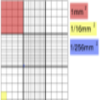Using the Haemocytometer

Age 14 to 16 Challenge Level:

Practise your skills of proportional reasoning with this interactive haemocytometer.Countdown

Age 7 to 14 Challenge Level:

Here is a chance to play a version of the classic Countdown Game.Fractions and Percentages Card Game

Age 11 to 16 Challenge Level:

Match the cards of the same value.